International
Tables for
Crystallography
Volume C
Mathematical, physical and chemical tables
Edited by E. Prince

International Tables for Crystallography (2006). Vol. C, ch. 4.3, pp. 413-414

## Section 4.3.5.3. Lattice direction oriented parallel to a direction (fibre texture)

B. B. Zvyaginn

#### 4.3.5.3. Lattice direction oriented parallel to a direction (fibre texture)

| top | pdf |

A fibre texture occurs when the crystals forming the specimen have a single direction in common. Each point of the reciprocal lattice describes a circle lying in a plane normal to the texture axis. The pattern, considered as plane sections of the reciprocal-lattice representation, resembles rotation diagrams of single crystals and approximates to the patterns given by cylindrical lattices (characteristic, for example, of tubular crystals).

If the a axis is the texture axis, the hk rods are at distances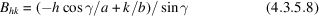from the texture axis and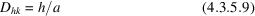from the plane normal to the texture axis (the zero plane b*c*). On rotation, they intersect the plane normal to the incident beam and pass through the texture axis in layer lines at distances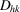from the zero line, while the reflection positions along these lines are defined by their distances from the textures axis (see Fig. 4.3.5.3):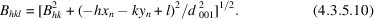Figure 4.3.5.3 | top | pdf |The projections of the reciprocal axes on the plane ab of the direct lattice, with indications of the distances B and D of the hk rows from the fibre-texture axes a or [hk].

If the texture axis forms an anglewith the a axis and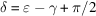with the projection of a* on the plane ab, then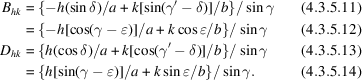The relation between the angles δ,, and the direction [hk] of the texture axis is given by the expression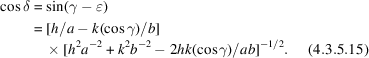The layer lines with constant h that coincide when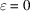are split when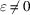according to the sign of k, since then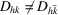andand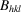defining the reflection positions along the layer line take other values. Such peculiarities have been observed by means of selected-area electron diffraction for tabular particles and linear crystal aggregates of some phyllosilicates in the simple case of γ = π/2 (Gritsaenko, Zvyagin, Boyarskaya, Gorshkov, Samotoin & Frolova, 1969).

When fibres or linear aggregates are deposited on a film (for example, in specimens for high-resolution electron diffraction) with one direction parallel to a plane, they form a texture that is intermediate between lamellar and fibre. The points of the reciprocal lattice are subject to two rotations: around the fibre axis and around the normal to the plane. The first rotation results in circles, the second in spherical bands of different widths, depending on the position of the initial point relative to the texture axis and the zero plane normal to it. The diffraction patterns correspond to oblique plane sections of reciprocal space, and consist of arcs having intensity maxima near their ends; in some cases, the arcs close to form complete circles. In particular, when the particle elongation is in the a direction, the angular range of the arcs decreases with h and increases with k (Zvyagin, 1967).

### References

Gritsaenko, G. S., Zvyagin, B. B., Boyarskaya, R. V., Gorshkov, A. I., Samotoin, N. D. & Frolova, K. E. (1969). Methods of electron microscopy of minerals. Moscow: Nauka.Google Scholar
Zvyagin, B. B. (1967). Electron diffraction analysis of clay mineral structures. New York: Plenum.Google Scholar